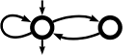Awali Another Weighted Automata library
ratexp.hh
Go to the documentation of this file.
1
2 // This file is part of Awali.
3 // Copyright 2016-2021 Sylvain Lombardy, Victor Marsault, Jacques Sakarovitch
4 //
5 // Awali is a free software: you can redistribute it and/or modify
6 // it under the terms of the GNU General Public License as published by
7 // the Free Software Foundation, either version 3 of the License, or
8 // (at your option) any later version.
9 //
10 // This program is distributed in the hope that it will be useful,
11 // but WITHOUT ANY WARRANTY; without even the implied warranty of
12 // MERCHANTABILITY or FITNESS FOR A PARTICULAR PURPOSE. See the
13 // GNU General Public License for more details.
14 //
15 // You should have received a copy of the GNU General Public License
16 // along with this program. If not, see <http://www.gnu.org/licenses/>.
17
18 #ifndef DYN_CORE_RATEXP_HH
19 #define DYN_CORE_RATEXP_HH
20
21 #include<memory>
25
26 namespace awali {
27 namespace dyn {
28
66 class ratexp_t : public std::shared_ptr<abstract_ratexp_t> {
67 public:
68
72  ratexp_t() : std::shared_ptr<abstract_ratexp_t>() {}
73
74
82  template<class T>
83  ratexp_t(const std::shared_ptr<T>& ptr,
84  typename std::enable_if<
86  int
87  >::type = 0)
88  : std::shared_ptr<abstract_ratexp_t>(ptr)
89  {}
90
109  ratexp_t(std::string str,
112  bool fixed_alphabet = true);
113
114
117
118  /* Concatenation of two rational expressions. */
119  ratexp_t operator* (ratexp_t exp);
120
124  return shared_ptr<abstract_ratexp_t>::operator*();
125  }
126
136  static ratexp_t from (std::string str,
137  std::string weightset = "B",
138  std::string alphabet ="auto");
139
150  static ratexp_t from_context (std::string str,
151  context_t ctx,
152  bool fixed_alphabet = true);
153
157  [[deprecated("Use constructor of ratexp_t instead")]]
158  static ratexp_t from_context(std::string str,
161  bool fixed_alphabet = true);
162
171  static ratexp_t from_range(std::string str,
172  int l,
173  int u,
174  std::string weightset = "B");
175
179  static ratexp_t from(std::string str, std::string weightset = "B");
180
184  static ratexp_t from_size(std::string str,
185  unsigned n,
186  std::string weightset = "B");
187  };
188
197  static ratexp_t from(std::string str,
198  unsigned n,
199  std::string weightset = "B");
200
204  static ratexp_t from(std::string str,
205  std::vector<std::string> alphabets,
206  std::string weightset ="B");
207  };
208
209 };
210
211 }} // end of namespaces awali::dyn, and awali
212 #endif
Abstract interface for rational expression at the dynamical layer; lists the services provided by aut...
Definition: abstract_ratexp.hh:91
Dynamical wrapper for a context, that is a weightset and a labelset.
Definition: context.hh:43
Main class for representing rational expresson at the dynamical layer.
Definition: ratexp.hh:66
ratexp_t operator+(ratexp_t exp)
Sum of two rational expressions.
ratexp_t operator*(ratexp_t exp)
ratexp_t(const std::shared_ptr< T > &ptr, typename std::enable_if< std::is_base_of< abstract_ratexp_t, T >::value, int >::type=0)
Builds a ratexp_t from a shared pointer to a class derived of abstract_ratexp_t; should generally not...
Definition: ratexp.hh:83
ratexp_t()
Buils an automaton_t that is essentially a nullptr; should generally not be used.
Definition: ratexp.hh:72
static ratexp_t from_size(std::string str, unsigned n, std::string weightset="B")
static ratexp_t from(std::string str, unsigned n, std::string weightset="B")
static ratexp_t from_range(std::string str, int l, int u, std::string weightset="B")
static ratexp_t from_context(std::string str, context::labelset_description ld, context::weightset_description wd, bool fixed_alphabet=true)
ratexp_t(std::string str, context::labelset_description ld, context::weightset_description wd, bool fixed_alphabet=true)
Builds the expression represented by str and whose labelset is described by ld and weightset by wd.
static ratexp_t from(std::string str, std::string weightset="B")
static ratexp_t from(std::string str, std::vector< std::string > alphabets, std::string weightset="B")
static ratexp_t from_context(std::string str, context_t ctx, bool fixed_alphabet=true)
Builds a rational expression from a string representation and a context.
static ratexp_t from(std::string str, std::string weightset="B", std::string alphabet="auto")
Builds a rational expression from its string representation.
std::shared_ptr< weightset_description_impl > weightset_description
Definition: description_types.hh:33
weightset_description weightset(const std::string &k)
std::shared_ptr< labelset_description_impl > labelset_description
Definition: description_types.hh:32
std::vector< std::vector< char > > alphabets(automaton_t tdc)
static constexpr TOP< void > value
Definition: priority.hh:93
Main namespace of Awali.
Definition: ato.hh:22
Helper class that contains convenience factories to build expressions whose labels are integers.
Definition: ratexp.hh:167
Helper class that contains convenience factories to build expressions whose labels are tuples.
Definition: ratexp.hh:193